# SAT II Math II : Quartiles and Interquartile Range

## Example Questions

### Example Question #1 : Quartiles And Interquartile Range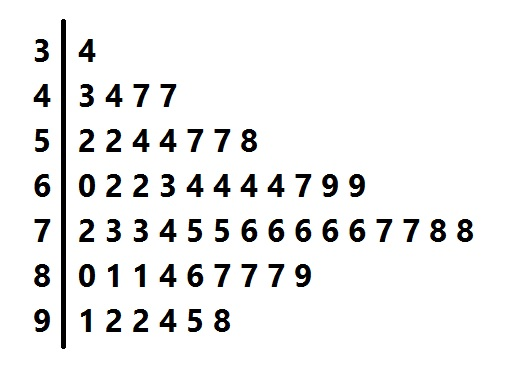Above is the stem-and-leaf display for a group of test scores. Give the interquartile range of the scores represented.

Possible Answers: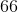Correct answer:Explanation:

The interquartile range is the difference between the third and first quartiles. To find these quartiles, first find the median of the scores. There are 53 scores represented, so look for the score in position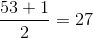.

As can be seen in this diagram, the score is 74.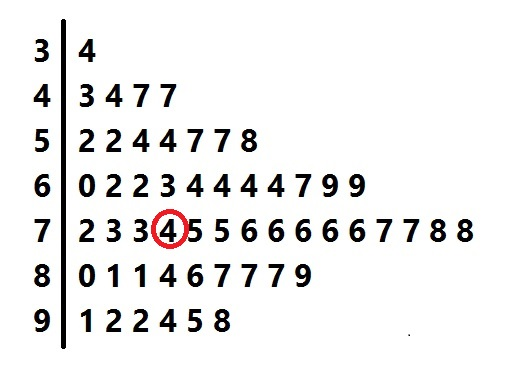The first quartile is the median of the lower half of the scores - that is, the lower 26 scores. Since 26 is even, the median is the mean of the scores in positions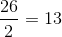and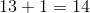.

As can be seen in this diagram, these scores are 60 and 62.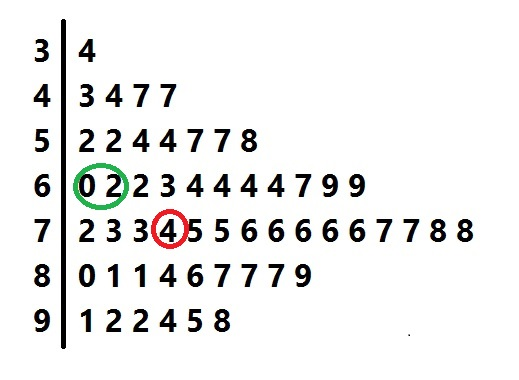The first quartile of the scores is therefore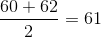The third quartile, similarly, can be found by finding the mean of the 13th and 14th elements in the top half of the scores: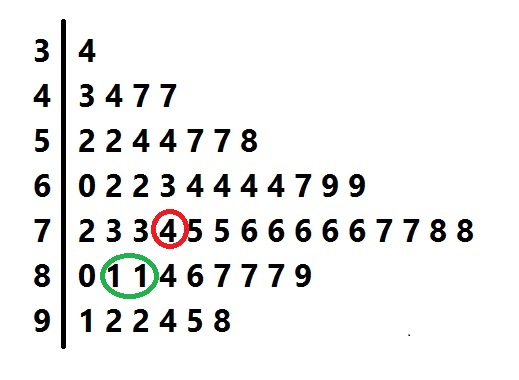As can be seen in this diagram, these scores are 81 and 81, so the third quartile is 81.

The interquartile range is the difference: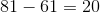### Example Question #1 : Quartiles And Interquartile RangeAbove is the stem-and-leaf display for a group of test scores. What score is at the third quartile?

Possible Answers: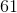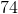Correct answer:Explanation:

To find the third quartile, first find the median of the scores. There are 53 scores represented, so look for the score in position.

As can be seen in this diagram, the score is 74.The third quartile is the median of the upper half of the scores - that is, the higher 26 scores. Since 26 is even, the median is the mean of the scores in positionsandfrom the top.As can be seen in this diagram, both of these scores are 81, so the third quartile is 81.

### Example Question #2 : Quartiles And Interquartile RangeAbove is the stem-and-leaf display for a group of test scores. What is the first quartile?

Possible Answers:Correct answer:Explanation:

To find the first quartile, first find the median of the scores. There are 53 scores represented, so, 53 being odd, look for the score in the center. This is the score in position.

As can be seen in this diagram, the score is 74.The first quartile is the median of the lower half of the scores - that is, the lower 26 scores. Since 26 is even, the median is the mean of the scores in positionsandfrom the bottom.

As can be seen in this diagram, these scores are 60 and 62.The first quartile of the scores is therefore.

### Example Question #4 : Quartiles And Interquartile Range

Consider the following numbers: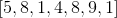.  What is the value of the first quartile?

Possible Answers:Correct answer:Explanation:

The first quartile is Q1.

Reorganize the numbers in chronological order.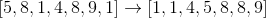The Q1 is the median of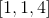, which is the three numbers left of the median of the entire set of numbers, 5.

The answer is:### All SAT II Math II Resources